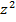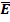# Suppose that the energy of a particle can be represented by the expression

Suppose that the energy of a particle can be represented by the expression

E(z)awhere zis a coordinate or momentum and can take

on all values from -infto inf.

(a) Show that the average energy per particle for a system of such

particles subject to Boltzmann statistics will bekT/2.

(b) State the principle of equipartition of energy and discuss briefly its

relation to the above calculation.